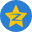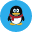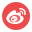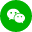# 已知某国在某核算期的有关数据如下(单位:亿本币):家庭消费支出为100,政府消费支出为20,家庭投资A.100+20+30+400+150+50+55=805

B.100+20+30+400+150+50-55=695

C.100+20+30+400+150=700

D.100+30+400+50-55=525

B